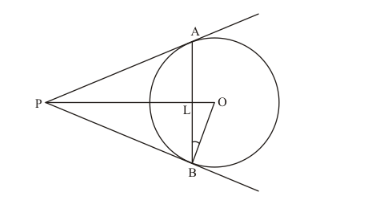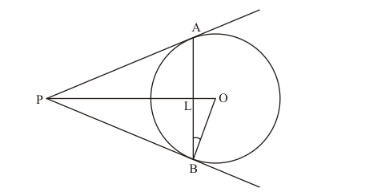# In the given figure, AB is a chord of length 16 cm of a circle of radius 10 cm.

Question:

In the given figure, AB is a chord of length 16 cm of a circle of radius 10 cm. The tangents at A and B intersect at a point P. Find the length of PA.Solution:

Considerand.From the property of tangents we know that the length of two tangents drawn form an external point will be equal. Therefore we have,

PA = PB

OB = OA (They are the radii of the same circle)

PO is the common side

Therefore, from SSS postulate of congruency, we have,Hence,…… (1)

Now considerand. We have,(From (1))

PA is the common side.

From the property of tangents we know that the length of two tangents drawn form an external point will be equal. Therefore we have,

PA = PB

From SAS postulate of congruent triangles, we have,

$\triangle P L A \cong \triangle P L B$

Therefore,

LA = LB

It is given that AB = 16. That is,

LA + LB = 16

LA + LA = 16

2LA = 16

LA = 8

LB = 8

Also, ALB is a straight line. Therefore,

$\angle A L B=180^{\circ}$

That is,

$\angle P L A+\angle P L B=180^{\circ}$

Since $\triangle P L A \cong \triangle P L B$,

$\angle P L A=\angle P L B$

Therefore,

$2 \angle P L B=180^{\circ}$

$\angle P L B=90^{\circ}$

Now let us consider. We have,

$O L^{2}=O B^{2}-O L^{2}$

$O L^{2}=10^{2}-8^{2}$

$O L^{2}=100-64$

$O L^{2}=36$

$O L=6$

Consider $\Delta O P B$ Here,

$\angle O B P=90^{\circ}$ (Since the radius of the circle will always be perpendicular to the tangent at the point of contact)

Therefore,

$P B^{2}=O P^{2}-O B^{2} \ldots \ldots(1)$

Now consider $\triangle P L B$

$P B^{2}=P L^{2}+L B^{2} \ldots \ldots(2)$

Since the Left Hand Side of equation (1) is same as the Left Hand Side of equation (2), we can equate the Right Hand Side of the two equations. Hence we have,

$O P^{2}-O B^{2}=P L^{2}+L B^{2}$.....(3)

From the figure we can see that,

OP = OL + LP

Therefore, let us replace OP with OL + LP in equation (3). We have,

$(O L+P L)^{2}-O B^{2}=P L^{2}+L B^{2}$

We have found that OL = 6 and LB = 8. Also it is given that OB = 10. Substituting all these values in the above equation, we get,

$(6+P L)^{2}-10^{2}=P L^{2}+8^{2}$

$36+P L^{2}+2 \times 6 \times P L-100=P L^{2}+64$

$12 P L=128$

$P L=\frac{32}{3}$

Now, let us substitute the value of $P L$ in equation (2). We get,

$P B^{2}=\left(\frac{32}{3}\right)^{2}+8^{2}$

$P B^{2}=\frac{1024}{9}+64$

$P B^{2}=\frac{1600}{9}$

$P B=\sqrt{\frac{1600}{9}}$

$P B=\frac{40}{3}$

We know that tangents drawn from an external point will always be equal. Therefore,

PB = PA

Hence, we have,

$P A=\frac{40}{3}$